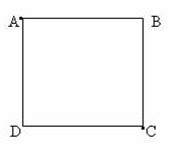## Sunday, March 06, 2011

### Multiple Choice Practice Questions on Electrostatics

“Live as if you were to die tomorrow. Learn as if you were to live for ever.”

Mahatma Gandhi

If you grasp the fundamental principles well, you will be able to answer multiple choice questions within the stipulated time. If you are confused about the fundamental principles, you will be tempted to waste your time while dealing with even simple questions just because of some simple distractions. See the following questions:

(1) A battery of emf 12 V is connected in series with two initially uncharged capacitors, each of value 100 μF (Fig.). The capacitors are fully charged. If the total energy spent by the battery for charging the capacitors is E joule, the energy of each capacitor is

(a) E

(b) E/2

(c) 2 E

(d) E/4

(e) E/8

If the total charge supplied by the battery is Q coulomb, the total energy E supplied by the capacitor is given by

E = VQ joule where V is the emf of the battery (which is 12 volt).

The two identical 100 μF capacitors in series is equivalent to a single capacitor of capacitance C = 50 μF. The energy of this charged capacitor combination is ½ CV2 joule = ½ VQ joule since Q = CV.

Therefore, the energy of the charged capacitor combination is ½ E.

Since there are two identical capacitors in the combination, the energy of each capacitor is E/4.

[So the capacitors have gained a total energy of E/2, even though the battery has delivered a total energy E. What happens to the other half of the energy? Well, it is irrecoverably lost as heat generated in the resistance of the circuit].(2) Charges of equal magnitude are fixed at the diagonally opposite corners A and C of a non conducting square frame ABCD (Fig.). In case (i) the charges at A and C are positive and a third free negative charge q is moved from B to D. In case (ii) the charges at A and C are negative and a third free negative charge q is moved from B to D. In case (iii) the charges at A and C are of opposite sign and a third free negative charge q is moved from B to D. Pick out the correct statement regarding the work done for moving the charge q:

(a) In case (i) the work done is positive

(b) In case (ii) the work done is positive

(c) In case (i) the work done is negative

(d) In case (iii) the work done is positive

(e) In all cases the work done is zero

In case (i) the potentials at B and D are positive, but of equal value. In case (ii) the potentials at B and D are negative, but of equal value. In case (iii) the potentials at B and D are zero. Therefore, in all cases the potential difference between points B and D is zero so that the work done in moving the charge q from B to D is zero.

You will find some useful questions (MCQ) with solution here.